# Measuring Mass Worksheet for Grade 3

Download Measuring Mass Worksheet for Grade 3Q.1) Convert:
6 kg = __ g

Q.2) Convert:
2 kg 186 g = __ g

Q.3) Convert:
4000 g = __ kg

Q.4) Convert:
2604 g = __ kg __ g

Q.5) Add 13 kg 136 g and 54 kg 641 g ?
a) 64 kg 558 g
b) 70 kg 643 g
c) 67 kg 777 g

Q.6) Add 25 kg 235 g and 12 kg 784 g ?
a) 38 kg 019 g
b) 34 kg 239 g
c) 41 kg 139 g

Q.7) Subtract:
46 kg 768 g – 21 kg 255 g ?
a) 25 kg 513 g
b) 22 kg 635 g
c) 23 kg 602 g

Q.8) Subtract:
63 kg 256 g – 32 kg 535 g ?
a) 27 kg 682 g
b) 28 kg 642 g
c) 30 kg 721 g

### Measuring Mass Worksheet Explanations

Q.1) Explanation – Measuring Mass Worksheet

As we know that,
1 kg = 1000 g
To find 6 kg in g. We have to multiply both sides by 6
( 6 x 1 ) kg = ( 6 x 1000 ) g
6 kg = 6000 g

Q.2) Explanation – Measuring Mass Worksheet

2 kg 186 g can also be written as 2 kg + 186 g
To change 2 kg + 186 g into g
we have to convert 2 kg in g
As we know that,
1 kg = 1000 g
2 kg = ( 2 x 1000 ) g
2 kg = 2000 g
Therefore,
2 kg + 186 g = 2000 g + 186 g
= 2186 g

Q.3) Explanation – Measuring Mass Worksheet

As we know that,
1 kg = 1000 g
or 1000 g = 1kg
then,
1 g = 1/1000 kg
To find 4000 g in kg. We have to multiply both sides by 4000
( 4000 x 1 ) g = ( 4000 x 1/1000 )kg
4000 g = 4 kg

Q.4) Explanation – Measuring Mass Worksheet

As we know that,
1 kg = 1000 g
or 1000 g = 1 kg
then,
1 g = 1/1000 kg

To find 2604 g in kg and g. We have to multiply both sides by 2604
( 2604 x 1 ) g = ( 2604 x 1/1000 )kg

2604 g = 2.604 kg
Digits before decimal point is written with kilogram ( kg ) and after decimal point with gram ( g )
Hence, 2604 g = 2 kg 604 g

Q.5) Explanation –

Step I: Write Kilograms (kg) and Grams (g) aligned in columns properly.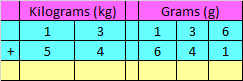Step II: Add Kilograms (kg) and Grams (g) as we add numbers in Addition.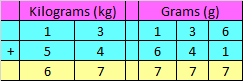Hence, the sum is 67 kg 777 g

Correct Answer – c) 67 kg 777 g

Q.6) Explanation – Measuring Mass Worksheet

Step I: Write Kilograms (kg) and Grams (g) aligned in columns properly.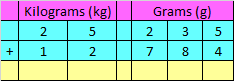Step II: Add Kilograms (kg) and Grams (g) as we add numbers in Addition.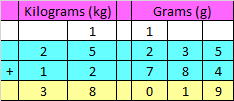Hence, the sum is 38 kg 019 g

Correct Answer – a) 38 kg 019 g

Q.7) Explanation –

Step I: Write Kilograms (kg) and Grams (g) aligned in columns properly.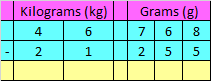Step II: Subtract Kilograms (kg) and Grams (g) as we subtract numbers in Subtraction.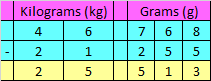Hence, the difference is 25 kg 513 g

Correct Answer – a) 25 kg 513 g

Q.8) Explanation – Measuring Mass Worksheet

Step I: Write Kilograms (kg) and Grams (g) aligned in columns properly.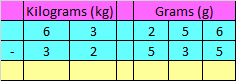Step II: Subtract Kilograms (kg) and Grams (g) as we subtract numbers in Subtraction.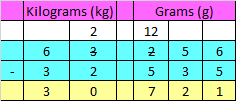Hence, the difference is 30 kg 721 g

Correct Answer – c) 30 kg 721 g